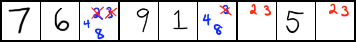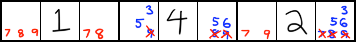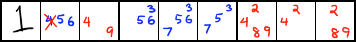# Naked Pairs, Naked Triples, Naked Quads Strategy

In sudoku, you will sometimes reach a point where you can't solve a square, but you can narrow down the possible numbers in that square, sometimes referred to as candidates. In some cases, you can make logical conclusions from those candidates that will help you solve the rest of the puzzle.

### Naked Pair

In sudoku, a naked pair is a set of exactly two candidates that are in exactly two squares in a row, column, or block. For example:In this example, the 2 and 3 in red on the right side is a naked pair. One of the squares in the pair has to be 2, and one of the squares in the pair has to be 3. If a square that is not part of the naked pair were set to 2, for example, then both of the squares in the naked pair would have 3 as the only candidate, which would be invalid.

Because of this, you can remove 2 and 3 as candidates from the other cells in the row. The 2 and 3 in the other cells have been crossed out in red in this example.

#### Interactive Example

The following interactive puzzle has several naked pairs that can be used to eliminate possible candidates. Can you find them?

Puzzle string:

If you are using Internet Explorer 8 or lower, or if you are using Android Browser 2 or lower, please upgrade to a newer web browser to play Thonky Sudoku.

### Naked Triple

Naked triples are harder to spot. Sometimes, all three numbers in a naked triple appear in all three squares of the triple. However, sometimes the numbers of the triple don't all appear in all three squares, as in the example below.The squares that have 7, 8, and 9 in red form a naked triple. Notice that if a square other than one of the naked triple squares were set to 7, for example, then the three naked triple squares would only have 8 and 9 as their candidates. The square that has 7, 8, and 9 in it would have both 8 and 9 as its remaining candidates, but each of the other two naked triple squares would be set to 8 and 9, respectively. This would be invalid, because the square with 8 and 9 as its candidates would no longer have any valid options.

Because of this, you can get rid of 7, 8, and 9 from the other cells in this row.

#### Interactive Example

The following interactive puzzle has several naked triples that can be used to eliminate possible candidates. Can you find them?

Puzzle string:

If you are using Internet Explorer 8 or lower, or if you are using Android Browser 2 or lower, please upgrade to a newer web browser to play Thonky Sudoku.

Naked quads are rare, but they can occur. They can be difficult to find, however, unless you specifically look for them.In the example above, the numbers in red form a naked quad. The four squares that contain the red numbers only contain numbers 2, 4, 8, and 9. That means that those four squares MUST contain those four numbers. Because of that, you can eliminate the 4 as shown crossed out in red.

#### Interactive Example

The following interactive puzzle has several naked quads that can be used to eliminate possible candidates. Can you find them?

Puzzle string:

If you are using Internet Explorer 8 or lower, or if you are using Android Browser 2 or lower, please upgrade to a newer web browser to play Thonky Sudoku.

### Example Puzzle

Try solving the following interactive puzzle. It can be solved using only the Naked Pairs, Triples, and Quads strategy.